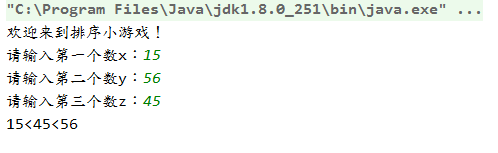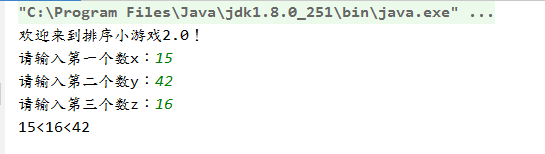• 2019-07-08 16:01:05
package jaz;

import java.util.Scanner;

public class sort {
public static void main(String[] args) {
Scanner sc = new Scanner(System.in);

System.out.println("输入num1");
int num1 = sc.nextInt();
System.out.println("输入num2");
int num2 = sc.nextInt();
System.out.println("输入num3");
int num3 = sc.nextInt();

int t = 0;
if(num1 > num2){
t = num1;
num1 = num2;
num2 = t;
}
if(num1 > num3){
t = num1;
num1 = num3;
num3 = t;
}
if(num2 > num3){
t = num2;
num2 = num3;
num3 = t;
}
System.out.println("排序");
System.out.println(num1);
System.out.println(num2);
System.out.println(num3);
}

}



运行结果：

输入num1
999
输入num2
99
输入num3
9
排序
9
99
999开发语言 算法
更多相关内容
• 读取三个整数并按升序对它们进行排序输入格式 共一行，包含三个整数。 输出格式 首先，将三个整数按升序顺序输出，每行输出一个整数。 然后，输出一个空行。 紧接着，将三个整数按原输入顺序输出，每行输出一个...

题目描述：
读取三个整数并按升序对它们进行排序。

输入格式
共一行，包含三个整数。

输出格式
首先，将三个整数按升序顺序输出，每行输出一个整数。

然后，输出一个空行。

紧接着，将三个整数按原输入顺序输出，每行输出一个整数。

数据范围
−100≤输入整数≤100

输入样例：
7 21 -14

输出样例：
-14
7
21

7
21
-14

解题代码：

import java.util.Scanner;

public class Main{
public static void main(String[] args){
Scanner in = new Scanner(System.in);
int a, b, c;
a = in.nextInt();
b = in.nextInt();
c = in.nextInt();
int a1 = a;
int b1 = b;
int c1 = c;
if(a1 > b1)
{
int t;
t = a1;
a1 = b1;
b1 = t;
}
if(b1 > c1)
{
int t;
t = c1;
c1 = b1;
b1 = t;
}
if(a1 > b1)
{
int t;
t = a1;
a1 = b1;
b1 = t;
}
System.out.println(a1);
System.out.println(b1);
System.out.println(c1);
System.out.println();
System.out.println(a);
System.out.println(b);
System.out.println(c);
}
}


题目链接： https://www.acwing.com/problem/content/description/665/

鸣谢： ***

展开全文• 今天小编就为大家分享一篇java输入数据(不确定),排序,并求最大值的方法，具有很好的参考价值，希望对大家有所帮助。一起跟随小编过来看看吧
• import java.util.*; public class test2 { public static void main(String[] args) { Scanner scan = new Scanner(System.in);...//初始化一整型数组，长度为你输入数字的个数 for (int i = 0; i <

import java.util.*;

public class test2 {

public static void main(String[] args) {

Scanner scan = new Scanner(System.in);

String[] str = scan.nextLine().split(" ");// 输入时以空格隔开数字

int[] num = new int[str.length];//初始化一个整型数组，长度为你输入数字的个数

for (int i = 0; i < str.length; i++) {

num[i] = Integer.parseInt(String.valueOf(str[i]));//将字符转换为int型再赋给整型数组

}

Arrays.sort(num);//升序排序

System.out.println(“从小到大输出：”);

for (int j = 0; j < num.length; j++) {

System.out.println(num[j]);//从小到大输出

}

System.out.println(“从大到小输出：”);

for (int m = num.length-1; m >=0; m–) {

System.out.println(num[m]);//从大到小输出

}

}

}

展开全文• 一个Java数学排序小程序，给定三个整数x,y,z，程序处理后让这三个数由小到大排列输出，代码编写思路：想办法把最小的数放到x上，先将x与y进行比较，如果x> y则将x与y的值进行交换，然后再用x与z进行比较，如果x> z则...
• ## Java输入三个整数 从小到大排列

千次阅读 多人点赞 2020-12-01 19:27:19
今天突然一想控制台输入三个数，如何将这三个数从小到大排序 经过段短暂的思考，直接上代码： public class Test04 { public static void main(String[] args) { demo01(); } /*7.题目：输入三个整数x,y,z，请把...

今天突然一想控制台输入三个数，如何将这三个数从小到大排序
经过短暂的思考，直接上代码：

public class Test04 {
public static void main(String[] args) {
demo01();
}
/*7.题目：输入三个整数x,y,z，请把这三个数由小到大输出。*/
public static void demo01(){
System.out.println("欢迎来到排序小游戏！");

Scanner scanner = new Scanner(System.in);
System.out.print("请输入第一个数x：");
int x = scanner.nextInt();
System.out.print("请输入第二个数y：");
int y=scanner.nextInt();
System.out.print("请输入第三个数z：");
int z=scanner.nextInt();
if(x>y) {
if(x>z){
if(y>z){
System.out.println(z+"<"+y+"<"+x);
return;
}else{
System.out.println(y+"<"+z+"<"+x);
return;
}
}else{
System.out.println(y+"<"+x+"<"+z);
return;
}
}
if(x>z){
if(z>y){
System.out.println(y+"<"+z+"<"+x);
return;
}else{
if(x>y){
System.out.println(z+"<"+y+"<"+x);
return;
}else{
System.out.println(z+"<"+x+"<"+y);
return;
}
}
}
if(y>z){
if(z>x){
System.out.println(x+"<"+z+"<"+y);
return;
}else{
if(x>y){
System.out.println(z+"<"+y+"<"+x);
return;
}else {
System.out.println(z+"<"+x+"<"+y);
return;
}
}
}
}
}


接下来我们看看运行结果：没有问题！Nice！
但是感觉代码有点繁琐，经过一段时间后想出来另外一种方法
--------------------------------------------------------------------------------------------------------
方法二

public class Test04 {
public static void main(String[] args) {
demo01();
}
public static void demo01(){
System.out.println("欢迎来到排序小游戏2.0！");

Scanner scanner = new Scanner(System.in);
System.out.print("请输入第一个数x：");
int x = scanner.nextInt();
System.out.print("请输入第二个数y：");
int y=scanner.nextInt();
System.out.print("请输入第三个数z：");
int z=scanner.nextInt();

if(x>y){
if(y>z){
System.out.println(z+"<"+y+"<"+x);
}else if(x>z){
System.out.println(y+"<"+z+"<"+x);
}else{
System.out.println(y+"<"+x+"<"+z);
}
}else{
if(z<x){
System.out.println(z+"<"+x+"<"+y);
}else if(y>z){
System.out.println(x+"<"+z+"<"+y);
}else{
System.out.println(x+"<"+y+"<"+z);
}
}
}
}


结果：代码比以前少了一半！起飞！

----------------------------------------------------------------------------------------------------------

方法三： 我giao，后来经过思考又发现了一个比较简单的写法，直接上代码：

/*7.题目：输入三个整数x,y,z，请把这三个数由小到大输出。*/
public static void demo07(){
System.out.println("欢迎来到排序小游戏！");

Scanner scanner = new Scanner(System.in);
System.out.print("请输入第一个数x：");
int x = scanner.nextInt();
System.out.print("请输入第二个数y：");
int y=scanner.nextInt();
System.out.print("请输入第三个数z：");
int z=scanner.nextInt();

/*获取最大值*/
int max=(x>y)? x:y;
max=(max>z)? max:z;
/*获取最小值*/
int min=(x<y)? x:y;
min=(min<z)? min:z;
/*获取中间值*/
int mid=x+z+y-max-min;
System.out.println(min+"<"+mid+"<"+max);

}


代码量又少一半，飞起！

展开全文• package zixue; import java.util.Scanner; //导包 public class BubbleSort { ... System.out.println("请输入5数进行排序："); int [] arr = new int; for(int i = 0;i < arr.length;i ++程序人生
• import java.util.Scanner;//比较大小public class Main3{public static void main(String[] args){input2 fnc=new input...System.out.println("输入第一个数字:");x=fnc.input();System.out.println("输入第二个数...
• Java由键盘输入三个整数，对他们排序并从小到大输出 Java由键盘输入三个整数分别存入变量mun1、mun2、mun3，对他们进行排序（使用if-else if--else），并且从小到大输出。开发语言 后端
• *题目：输入三个整数x,y,z，请把这三个数由小到大输出。 */ public class lianxi15 { public static void main(String[] args) { @SuppressWarnings("resource") Scanner sc=new Scanner(System.in); int ...
• ## Java三个数排序

千次阅读 2019-01-07 17:45:39
/* 要求： 输入： ... 请输入3个数,以空格隔开 ... 你输入三个数为 i=29,j=22,k=21 从小到大排序之后的值为： 你输入三个数为 i=21,j=22,k=29 */ import java.util.Scanner; ...入门
• Java设计一个程序，比较3个数的大小，按照从小到大的顺序输出 示例代码： public class Employee { public static void ...System.out.println(“请输入三个数”); int num1=scanner.nextInt(); int num2=scanner.n
• import java.util.Arrays; import java.util....//三角形边 int arr []=new int ; public void infor() { Scanner input = new Scanner(System.in); System.out.println("请输入三角形的三条边"); for(i.
• java 输入个数字,反转输出这个数字的值(实现方法)如下所示：package 第四天;import java.util.Scanner;public class 数字反转 {public static void main(String[] args) {Scanner sc = new Scanner(System.in);...
• 键盘输入三个数，实现从小到大排序，键盘输入从小到大// 导包import java.util.Scanner;public class Sort {public static void main(String[] args) {// 创建键盘输入对象Scanner sc = new Scanner(System.out....
• 用户输入三个整数，之后按照整数的大小从小到大输出
• import java.util.Scanner; public class Text{ public static void main(String[] args){ Scanner sc=new Scanner(System.in); int num1=sc.nextInt(); int num2=sc.nextInt(); int num3=sc.nextInt(); ...
• 使用冒泡排序实现： package test; import java.util.Scanner; public class Paixu { public static void main(String[] args) { //定义一int类型的数组 int[] array=new int; Scanner scan= ...
• Java数组大基本排序 1、冒泡排序 2、选择排序 3、插入排序 个人思路，大家互相参考，讨论 排序是把一堆数组有小到大、由大到小从头到尾按照一定的顺序排列起来的。 1、冒泡排序 冒泡排序指数组中的两相比，...
• 题目：输入三个整数x,y,z，请把这三个数由小到大输出。 2.解析 两两之间比较，选出最小数，保证num3的值最大，再比较剩余的数，将较大者的值赋给num2，排出顺序。 3.解决问题 代码如下： import java.util.Scanner; ...
• import java.util.Scanner; public class Demo5 { public static void main(String[] args) { Scanner input=new Scanner(System.in); System.out.println("请输入第一数："); int a=input.nextInt(); ...
• 上课上的有点快，今天主讲了一个排序的程序，就是输入三个数字进行排序，使用了if——else语句。我创建了一个包com.mxl.array，然后创建了两个的类。 package com.mxl.array; public class Number {  void...
• 优质文章，及时送达作者 | 小蓝来源 | 蓝桥(id:lanqiaocenter)排序算法可以分为两大类：1、非线性时间比较类排序：通过比较来决定元素间的相对次序，由于其时间复杂度不能突破O(nlogn)，因此称为非线性时间比较类...
• 输入一串字符，包含数字[0-9]和小写字母[a-z]，要求按数字从小到大、字母从a到z排序，并且所有数字排在字母后面 输入描述: 一串字符 输出描述: 按规则排序的一串字符 示例1 输入 a31bz 输出 abz13 ...
• import java.util.Scanner; public class AshuzuXz_b8_1 { public static void main(String[] args) { int[] array = new int;... System.out.println("请输入10数："); Scanner scanner ...
• 编写一个程序,输入a,b,c三个值,按从小到大...Console.WriteLine("请输入三个数");a=ic语言:任意输入两个数um1,unm2按从小到大的顺序排序输出额,这个问题是个很简单的问题,只有2个数字而已,只要一个比较就行了啊.#in...
• Python 对输入数字进行排序的方法要求，输入一串数字，并以列表的形式打印出来。number = input('请输入一串数字：')print(number)print(type(number))假设输入12345，得到结果如下：请输入一串数字:1234512345...
• 需求：由键盘输入三个整数分别存入变量num1、num2、num3，对它们进行排序, 并且从小到大输出 可以使用2种方法实现：一、使用if-else分支结构；二、使用java内置模块Arrays的排序方法。
• 今天做的这题主要用了Scanner类来获取输入数字，使用冒泡法来对数组进行排序，使用for循环来实现冒泡法和遍历数组。 一.Scanner类 我们可以通过 Scanner 类来获取用户的输入。（记得导入Scanner类的包java.util....算法
• 题目：输入三个整数 x,y,z，请把这三个数由小到大输出。 1.程序分析：我们想办法把最小的数放到 x 上，先将 x 与 y 进行比较，如果 x> y 则将 x 与 y 的值进行交换， 然后再用 x 与 z 进行比较，如果 x> z...算法...

# java输入三个数字排序java 订阅